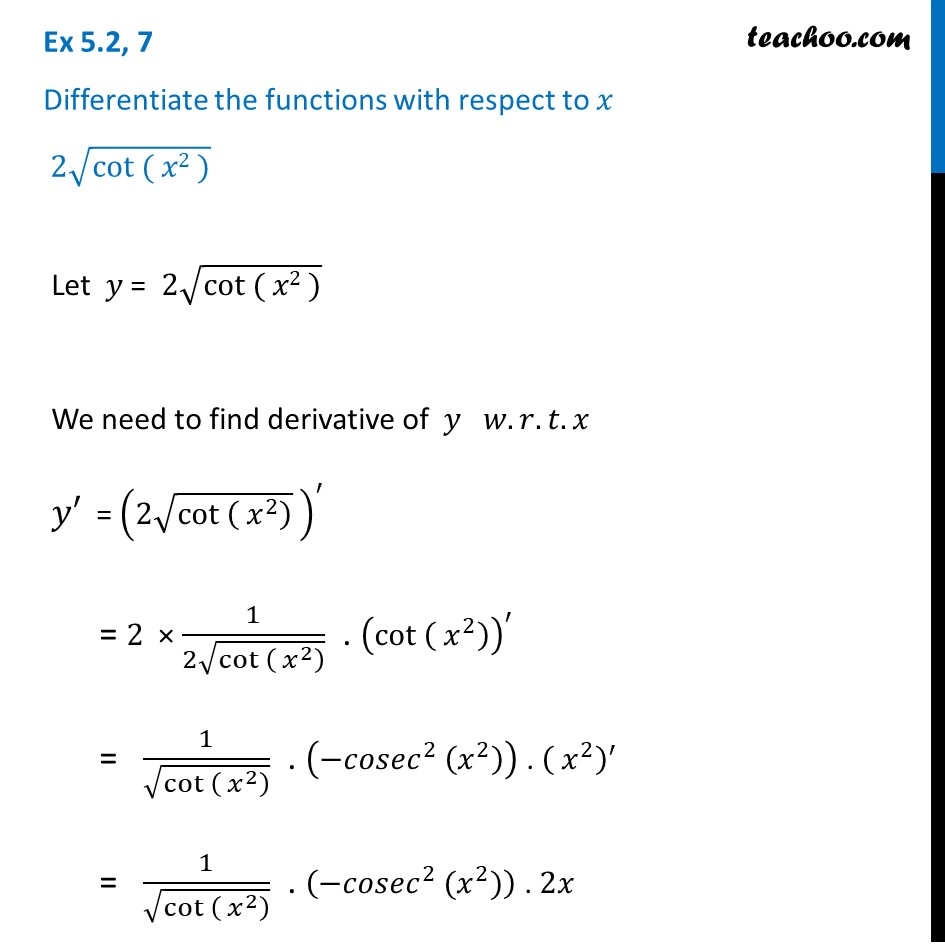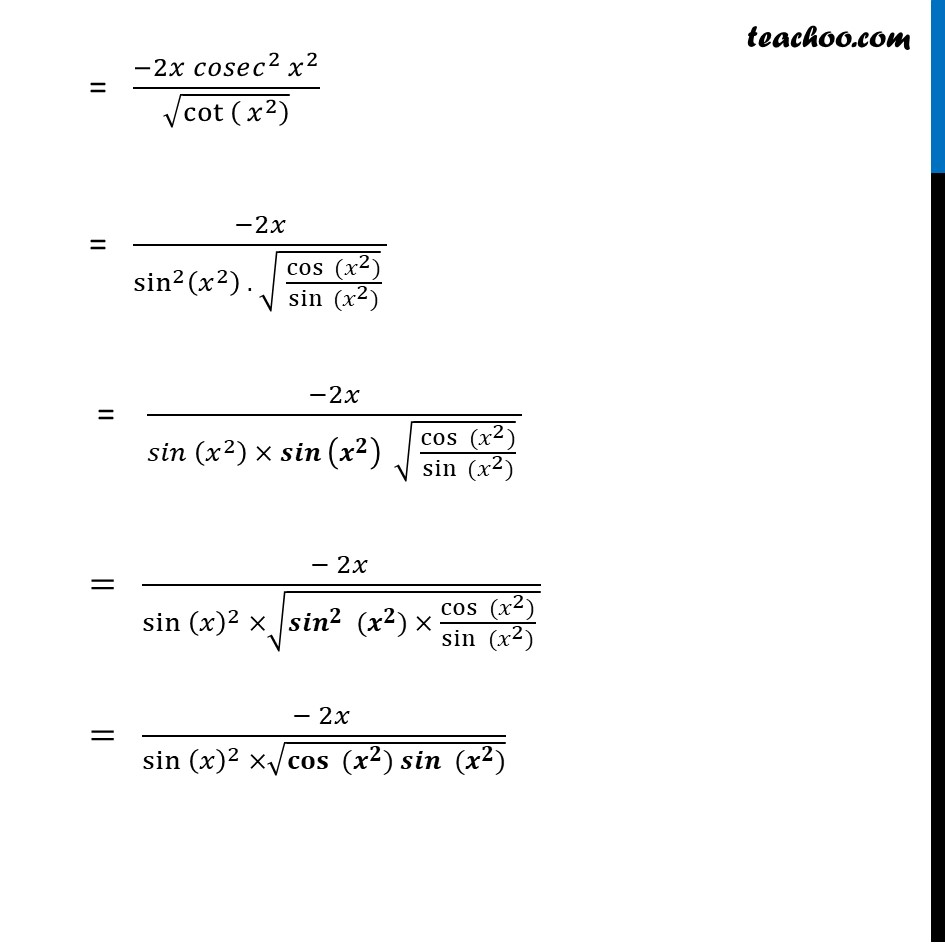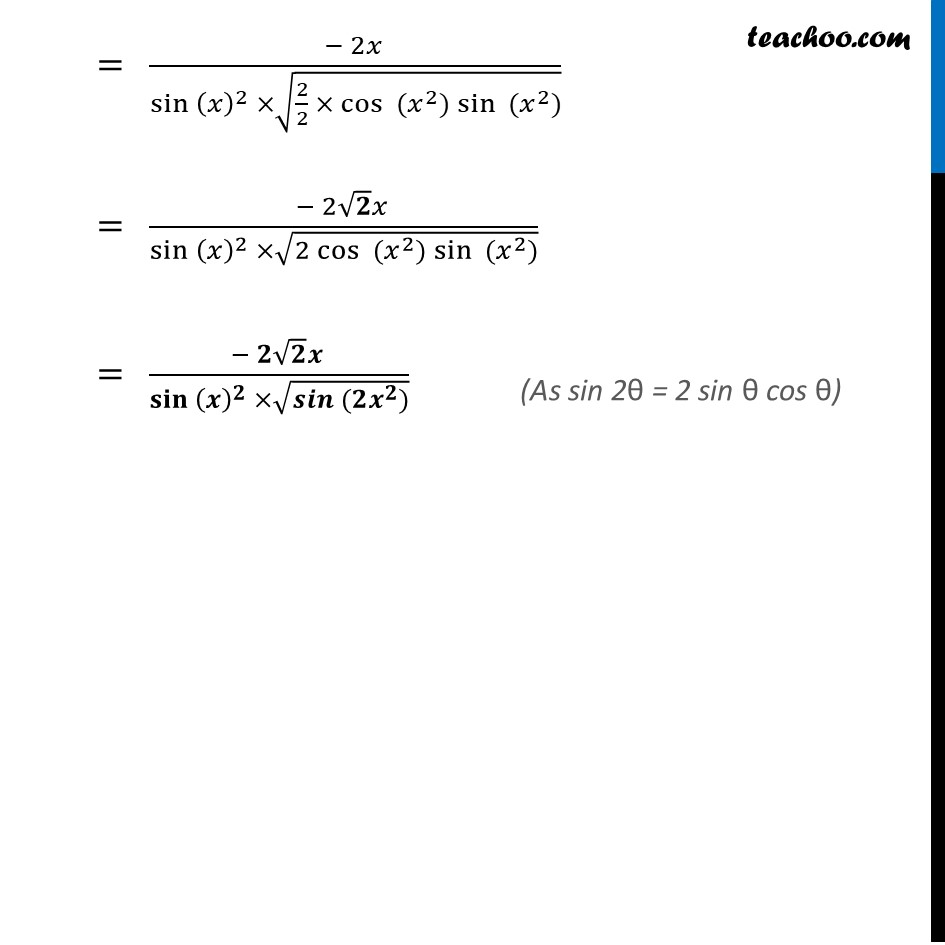Finding derivative of a function by chain rule

Chapter 5 Class 12 Continuity and Differentiability (Term 1)
Concept wise### Transcript

Ex 5.2, 7 Differentiate the functions with respect to 𝑥 2√(cot ( 𝑥2 ))Let 𝑦 = " " 2√(cot ( 𝑥2 )) We need to find derivative of 𝑦 𝑤.𝑟.𝑡.𝑥 𝑦′ = (2√(cot ( 𝑥^2 ) ) )^′ = 2 × 1/(2√(cot ( 𝑥^2 ) )) . (cot ( 𝑥^2 ))^′ = 1/√(cot ( 𝑥^2 ) ) . (−𝑐𝑜𝑠𝑒𝑐^2 (𝑥^2 )) . ( 𝑥^2 )^′ = 1/√(cot ( 𝑥^2 ) ) . (−𝑐𝑜𝑠𝑒𝑐^2 (𝑥^2)) . 2𝑥 = (−2𝑥" " 𝑐𝑜𝑠𝑒𝑐^2 𝑥^2)/√(cot ( 𝑥^2 ) ) = (−2𝑥)/(sin^2⁡(𝑥^2 ) . √( 〖cos 〗⁡〖(𝑥^2)〗/〖sin 〗⁡〖(𝑥^2)〗 ) ) "= " (−2𝑥)/(〖𝑠𝑖𝑛 〗⁡(𝑥^2 ) × 〖𝒔𝒊𝒏 〗⁡(𝒙^𝟐 ) √( 〖cos 〗⁡〖(𝑥^2)〗/〖sin 〗⁡〖(𝑥^2)〗 ) ) " " = (− 2𝑥 )/(〖sin 〗⁡〖(𝑥)^2 〗 ×√(〖〖𝒔𝒊𝒏〗^𝟐 〗⁡〖(𝒙^𝟐)〗 × 〖cos 〗⁡〖(𝑥^2)〗/sin⁡〖 (𝑥^2)〗 )) = (− 2𝑥 )/(〖sin 〗⁡〖(𝑥)^2 〗 ×√(〖𝐜𝐨𝐬 〗⁡〖(𝒙^𝟐)〗 𝒔𝒊𝒏⁡〖 (𝒙^𝟐)〗 )) = (− 2𝑥 )/(〖sin 〗⁡〖(𝑥)^2 〗 ×√(2/2 × 〖cos 〗⁡〖(𝑥^2)〗 sin⁡〖 (𝑥^2)〗 )) = (− 2√𝟐 𝑥 )/(〖sin 〗⁡〖(𝑥)^2 〗 ×√(2 〖cos 〗⁡〖(𝑥^2)〗 sin⁡〖 (𝑥^2)〗 )) = (− 𝟐√𝟐 𝒙 )/(〖𝐬𝐢𝐧 〗⁡〖(𝒙)^𝟐 〗 ×√(〖𝒔𝒊𝒏 〗⁡〖(𝟐𝒙^𝟐)〗 )) (As sin 2θ = 2 sin θ cos θ)